# Converting Text Representing Minutes and Seconds into Numerical Values in Microsoft Excel 2010

To convert the text representing minutes and seconds into numerical values, we use “LEFT”, “FIND”, “LEN” and “MID” functions in Microsoft Excel 2010.

LEFT: Returns the first character(s) in a text string based on the number of characters specified.

Syntax of “LEFT” function:            =LEFT (text,[num_chars])

Example: Cell A1contains the text “Broncho Billy Anderson”

=LEFT (A1, 7), and function will return “Broncho”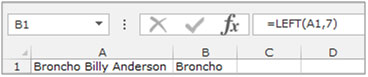FIND: This function returns the number of the character at which a specific character or text string is first found, reading left to right (not case-sensitive).

Syntax of “FIND” function:           =FIND (find_text,within_text,[start_num])

Example: Cell A1 contains the text “Broncho Billy Anderson”

=FIND ("Billy", A1, 1), andfunction will return 9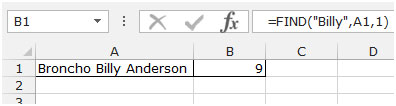MID:Return a specific number of character(s) from a text string, starting at the position specified based on the number of characters specified.

Syntax of “MID” function: =MID (text,start_num,num_chars)

Example: Cell A2 contains the text “Broncho Billy Anderson”

We want to return the Middle name from the cell A1.

Follow below given steps:

• Write the formula in cell B1.
• =MID (A1, 8, 7)
• Press Enter on your keyboard.
• The function will return “Billy”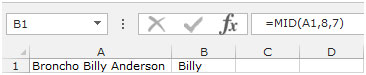LEN: Returns the number of characters in a text string.

Syntax of “LEN” function:             =LEN (text)

Example: Cell A1contains the text “Broncho Billy Anderson”

=LEN (A1), and function will return 22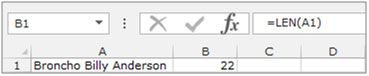Let’s take an example to understand how we can convert text representing minutes and seconds into numerical values.

Column A contains text string representing the time value in the “XmYs” format. X represent the number of minutes and Y represents the number of seconds.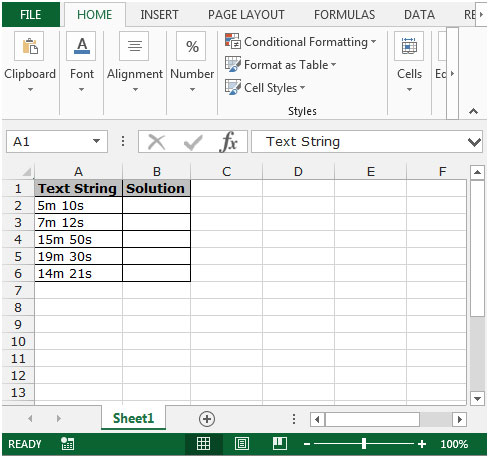We want to calculate the total number of seconds represented by each string in column A.

Follow below given steps:-

• Write the formula in cell B2.
• =(LEFT(A2,FIND("m",A2)-1)*60)+LEFT(MID(A2,FIND(" ",A2)+1,99),LEN(MID(A2,FIND(" ",A2)+1,99))-1)
• Press Enter on your keyboard.
• The function will return the total number of seconds.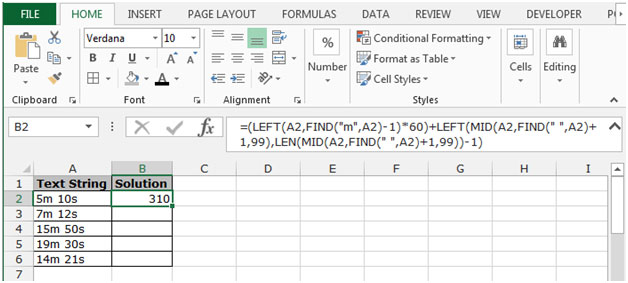• Copy the same formula by pressing the key Ctrl+C and paste in the range B3:B6 by pressing the key Ctrl+V on your keyboard.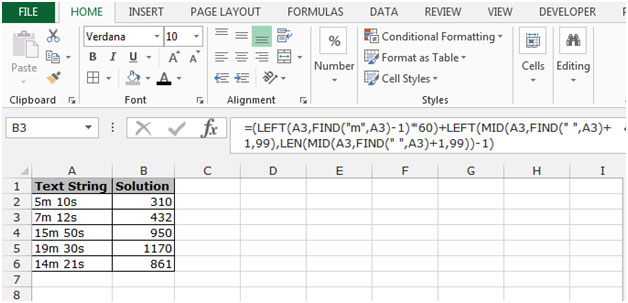This is the way we can convert the text that represents minutes and seconds into numerical values in Microsoft Excel.

1.